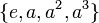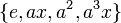# Fusion systems for dihedral group:D8

View fusion systems for particular groups | View other specific information about dihedral group:D8
There are multiple conventions on whether the term fusion system should refer to saturated fusion system or to a weaker notion. For our purposes, we will mostly be interested in saturated fusion systems, because these mimic/generalize situations where the$p$-group is a$p$-Sylow subgroup of some finite group. Unless otherwise specified, we are referring to saturated fusion systems when we talk about fusion systems.

This article discusses the possible fusion systems for the dihedral group of order eight.$G = \langle a,x \mid a^4 = x^2 = e, xax^{-1} = a^{-1} \rangle$.

There are, up to isomorphism, three possible fusion systems on$G$.

## Summary

Item Value
Total number of saturated fusion systems on a concrete instance of the group (strict, not up to isomorphism of fusion systems) 4
Total number of saturated fusion systems up to isomorphism 3
List of saturated fusion systems with orbit sizes inner fusion system (orbit size 1 under isomorphisms), non-inner non-simple fusion system for dihedral group:D8 (orbit size 2 under isomorphisms), simple fusion system for dihedral group:D8 (orbit size 1)
Number of simple fusion systems 1
Number of maximal saturated fusion systems, i.e., saturated fusion systems not contained in bigger saturated fusion systems 1 (the simple fusion system for dihedral group:D8)

## Family contexts

Family name Parameter values General information on fusion systems for family
dihedral group (of degree, order powers of 2) degree$n = 4$, order$2n = 8$ fusion systems for dihedral groups
For more on comparison with other groups of order 8, see fusion systems for groups of order 8.

## Description of saturated fusion systems

FACTS TO CHECK AGAINST FOR FUSION SYSTEMS:
For an abelian group of prime power order: identity functor controls strong fusion for saturated fusion system on abelian group|classification of saturated fusion systems on abelian group of prime power order

Isomorphism type of fusion system Number of such fusion systems under strict counting Can the fusion system be realized using a Sylow subgroup of a finite group? Does the identity functor control strong fusion? This would mean that all fusion occurs in the normalizer Is the fusion system simple? Smallest size embedding realizing this fusion system (if any)
inner fusion system 1 Yes Yes inner fusion system is not simple as a subgroup of itself
non-inner non-simple fusion system for dihedral group:D8 2 Yes No No D8 in S4
simple fusion system for dihedral group:D8 1 Yes No Yes D8 in PSL(3,2)
Total (3 rows) 4 -- -- -- --

## The inner fusion system: the fusion system obtained from inner automorphisms

This is the fusion system where all morphisms are obtained as the restriction of inner automorphisms of$G$. The isomorphisms are as follows.

Equivalence class under isomorphisms Subgroups involved Order Index Total number of subgroups Are all group automorphisms of each subgroup included? Size of automorphism group from the fusion system Total number of isomorphisms (including automorphisms and others) = (number of automorphisms)$\times$$\mbox{(number of subgroups)}^2$
trivial subgroup 1 8 1 Yes 1 1$\{ e, a^2 \}$ center of dihedral group:D8 2 4 1 Yes 1 1$\{ e, x \}, \{ e, a^2x \}$ non-normal subgroups of dihedral group:D8 (some of them) 2 4 2 Yes 1 4$\{ e, ax \}, \{ e, a^3x \}$ non-normal subgroups of dihedral group:D8 (some of them) 2 4 2 Yes 1 4$\{ e, a, a^2, a^3 \}$ cyclic maximal subgroup of dihedral group:D8 4 2 1 Yes 2 2$\{ e, x, a^2, a^2x \}$ Klein four-subgroups of dihedral group:D8 (one of them) 4 2 1 No 2 2$\{ e, ax, a^2, a^3x \}$ Klein four-subgroups of dihedral group:D8 (one of them) 4 2 1 No 2 2
whole group 8 1 1 No 4 4

### Sylow subgroups realizing this fusion system

This fusion system is realized by a group having dihedral group:D8 as its 2-Sylow subgroup if and if it possesses a normal complement, so the 2-Sylow subgroup is a retract of the group and the group is a semidirect product of a normal$p'$-subgroup and the dihedral group, or equivalently the group is a 2-nilpotent group.

Some examples are below:

Group Order Isomorphism class of normal complement Is it a direct product?
direct product of D8 and Z3 24 cyclic group:Z3 Yes
dihedral group:D24 24 cyclic group:Z3 No
SmallGroup(24,8) 24 cyclic group:Z3 No
direct product of D8 and Z5 40 cyclic group:Z5 Yes
dihedral group:D40 40 cyclic group:Z5 No

## Non-inner non-simple fusion system: center identified with one other conjugacy class

Further information: non-inner non-simple fusion system for dihedral group:D8

### Explicit description of fusion system

This fusion system is realized, for instance, in the symmetric group of degree four. In fact, the symmetric group of degree four is in essence the only example -- any example admits this as a subquotient. In the table below, we describe the subgroups explicitly using this realization, i.e., in terms of D8 in S4.

Equivalence class under isomorphisms, explicit description of subgroups Description of subgroups as permutation groups Subgroups involved Order Index Number of conjugacy classes of subgroups fused Total number of subgroups (=1 iff weakly closed subgroup for the fusion system) Are all group automorphisms of each subgroup included? Size of automorphism group from the fusion system Total number of isomorphisms (including automorphisms and others) = (number of automorphisms)$\times$ (number of subgroups)${}^2$
trivial subgroup$()$ 1 8 1 1 Yes 1 1$\{ e, a^2 \}, \{ e, ax \}, \{ e, a^3x \}$$\{ (), (1,3)(2,4)\}$,$\{ (), (1,4)(2,3) \}$,$\{ (), (1,2)(3,4) \}$ center of dihedral group:D8, non-normal subgroups of dihedral group:D8 (one of the two conjugacy classes) 2 4 2 3 Yes 1 9$\{ e, x \}, \{ e, a^2x \}$$\{ (), (1,3) \}, \{ (), (2,4) \}$ non-normal subgroups of dihedral group:D8 (one of the two conjugacy classes) 2 4 1 2 Yes 1 4$\{ e, a, a^2, a^3 \}$$\{ (), (1,2,3,4), (1,3)(2,4), (1,4,3,2) \}$ cyclic maximal subgroup of dihedral group:D8 4 2 1 1 Yes 2 2$\{ e, x, a^2, a^2x \}$$\{ (), (1,3), (1,3)(2,4), (2,4) \}$ Klein four-subgroups of dihedral group:D8 (one of them) 4 2 1 1 No (see below) 2 2$\{ e, ax, a^2, a^3x \}$$\{ (), (1,4)(2,3), (1,3)(2,4), (1,2)(3,4) \}$ Klein four-subgroups of dihedral group:D8 (one of them) 4 2 1 1 Yes 6 6
whole group 8 1 1 1 No (see below) 4 4
Total -- -- -- -- 8 10 -- -- --

Here is a description of the automorphism group from the fusion system in the two cases that it is not the full automorphism group:

• For the group$\{ e, x, a^2, a^2x \}$, which is one of the Klein four-subgroups of dihedral group:D8, the automorphisms arising from the fusion system are the identity map and the automorphism that interchanges$(1,3)$ with$(2,4)$, while fixing$()$ and$(1,3)(2,4)$.
• For the whole group, the automorphisms arising from the fusion system are precisely the inner automorphisms. This is true for all fusion systems on dihedral group:D8 because the outer automorphism group of this group is a 2-group, so it has no 2'-automorphisms.

### Realization in groups

Here are some examples of a group having dihedral group:D8 as a 2-Sylow subgroup such that this is the fusion system induced:

Group Order Dihedral group:D8 as a subgroup in this group Comment
symmetric group:S4 24 D8 in S4 this is the canonical, minimal example
symmetric group:S5 120 D8 in S5 the fusion system for this is precisely the same as that for D8 in S4 viewing symmetric group:S4 as an intermediate subgroup.

## Simple fusion system

Further information: simple fusion system for dihedral group:D8

Below is a description of the unique simple fusion system on this group.

### Explicit description of fusion system

Equivalence class under isomorphisms, explicit description of subgroups Subgroups involved Order Index Number of conjugacy classes of subgroups fused Total number of subgroups (=1 iff weakly closed subgroup for the fusion system) Are all group automorphisms of each subgroup included? Size of automorphism group from the fusion system Total number of isomorphisms (including automorphisms and others) = (number of automorphisms)$\times$$\mbox{(number of subgroups)}^2$
trivial subgroup 1 8 1 1 Yes 1 1$\{ e, a^2 \}$$\{ e, x \}, \{ e, a^2x \}$$\{ e, ax \}, \{ e, a^3x \}$,
center of dihedral group:D8, non-normal subgroups of dihedral group:D8 (both conjugacy classes) 2 4 3 5 Yes 1 25$\{ e, a, a^2, a^3 \}$ cyclic maximal subgroup of dihedral group:D8 4 2 1 1 Yes 2 2$\{ e, x, a^2, a^2x \}$ Klein four-subgroups of dihedral group:D8 (one of them) 4 2 1 1 Yes 6 6$\{ e, ax, a^2, a^3x \}$ Klein four-subgroups of dihedral group:D8 (one of them) 4 2 1 1 Yes 6 6
whole group 8 1 1 1 No (see below) 4 4
Total -- -- 8 10 -- -- --

For the whole group, the only automorphisms that are included in the fusion system are the inner automorphisms. This can be traced to the fact that the outer automorphism group of the dihedral group is 2-group, and in particular has no 2'-automorphisms.

### Realization in groups

Group Order Dihedral group:D8 as a subgroup of this group Comment
projective special linear group:PSL(3,2) (same as PSL(2,7)) 168 D8 in PSL(3,2) example of smallest order, but not the only minimal example
alternating group:A6 360 D8 in A6 also a minimal example